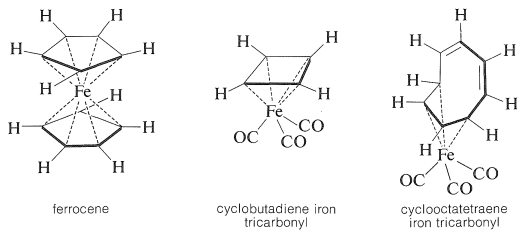# 27.7: Mössbauer Spectroscopy

$$\newcommand{\vecs}{\overset { \rightharpoonup} {\mathbf{#1}} }$$ $$\newcommand{\vecd}{\overset{-\!-\!\rightharpoonup}{\vphantom{a}\smash {#1}}}$$$$\newcommand{\id}{\mathrm{id}}$$ $$\newcommand{\Span}{\mathrm{span}}$$ $$\newcommand{\kernel}{\mathrm{null}\,}$$ $$\newcommand{\range}{\mathrm{range}\,}$$ $$\newcommand{\RealPart}{\mathrm{Re}}$$ $$\newcommand{\ImaginaryPart}{\mathrm{Im}}$$ $$\newcommand{\Argument}{\mathrm{Arg}}$$ $$\newcommand{\norm}{\| #1 \|}$$ $$\newcommand{\inner}{\langle #1, #2 \rangle}$$ $$\newcommand{\Span}{\mathrm{span}}$$ $$\newcommand{\id}{\mathrm{id}}$$ $$\newcommand{\Span}{\mathrm{span}}$$ $$\newcommand{\kernel}{\mathrm{null}\,}$$ $$\newcommand{\range}{\mathrm{range}\,}$$ $$\newcommand{\RealPart}{\mathrm{Re}}$$ $$\newcommand{\ImaginaryPart}{\mathrm{Im}}$$ $$\newcommand{\Argument}{\mathrm{Arg}}$$ $$\newcommand{\norm}{\| #1 \|}$$ $$\newcommand{\inner}{\langle #1, #2 \rangle}$$ $$\newcommand{\Span}{\mathrm{span}}$$$$\newcommand{\AA}{\unicode[.8,0]{x212B}}$$

A different form of molecular excitation is that of changes in the energies of the atomic nuclei. In general, enormous energies are involved, and such excitations will not be of interest to the study of organic chemistry unless the atomic energy levels are detectably influenced by the chemical surroundings of the nuclei. Usually this is not so, but there is one form of nuclear spectroscopy, known as Mössbauer spectroscopy, which is capable of giving chemical information. The technique would be used widely if there were more nuclei with the proper nuclear properties. For organic chemistry, probably the most important available nucleus is the iron nuclide $$\ce{^{57}Fe}$$ ($$2.2\%$$ of the natural mixture of iron isotopes). Iron occurs in many biologically important substances, such as hemoglobin, myoglobin, cytochromes, the iron storage substance ferritin, and so on, and there are a number of other types of stable organoiron compounds including ferrocene, cyclobutadiene iron tricarbonyl, and cyclooctatetraene iron tricarbonyl, which will be discussed in Chapter 31. These compounds present unusually difficult problems in how to formulate the bonding between carbon and iron. Important information has been obtained for such substances by Mössbauer spectroscopy.The essence of the Mössbauer technique as applied to $$\ce{^{57}Fe}$$ follows. A radioactive $$\ce{^{57}Co}$$ nucleus captures an electron and is converted to an excited $$\ce{^{57}Fe}$$ nucleus, which then emits a $$\gamma$$ ray and becomes an ordinary $$\ce{^{57}Fe}$$ nucleus. If the excited $$\ce{^{57}Fe}$$ nucleus is in a ridig material so that there is no recoil motion associated with the emission of the $$\gamma$$ ray, then this ray is extraordinarily monochromatic (has a very small $$\Delta \nu$$, Section 27-1) even though of great energy $$\left( 14.4 \: \text{KeV} = 3.3 \times 10^5 \: \text{kcal mol}^{-1} \right)$$. When such a $$\gamma$$ ray passes through a sample containing $$\ce{^{57}Fe}$$ atoms (also held rigidly), the $$\gamma$$ ray can be absorbed to produce another excited $$\ce{^{57}Fe}$$ nucleus. The chemical environment of the iron atoms can change the wavelength at which this absorption occurs. The problem is how to vary the wavelength of the $$\gamma$$ rays to match the nuclear absorption frequency. The way this is done is almost unbelievably simple - move the sample back and forth a few $$\text{mm sec}^{-1}$$ in the path of the $$\gamma$$ rays and measure the velocities at which absorption takes place. The velocity of light is $$3 \times 10^{11} \: \text{mm sec}^{-1}$$. Therefore, a Doppler effect of $$1 \: \text{mm sec}^{-1}$$ corresponds to a difference of only one par in $$3 \times 10^{11}$$. However, the selectivity of the recoilless $$\gamma$$ rays emitted from excited $$\ce{^{57}Fe}$$ nuclei is on the order of one part in $$5 \times 10^{+13}$$ (equivalent to about a $$7$$-$$\text{cm}$$ variation in the distance from the earth to the sun!).

A Mössbauer spectrum that has helped to corroborate the structure of cyclooctatetraene iron tricarbonyl is shown in Figure 27-12. The separation of the two absorption peaks in Figure 27-12 corresponds to a sample Doppler velocity of $$1.23 \: \text{mm sec}^{-1}$$. This Doppler effect means that there is the very small energy difference of $$1.4 \times 10^{-6} \: \text{kcal mol}^{-1}$$ in the two transitions shown.Figure 27-12: Mössbauer spectrum of cyclooctatetraene iron tricarbonyl in octane at $$78^\text{o} \text{K}$$. Notice that a spread of Doppler velocities of about $$3 \: \text{mm sec}^{-1}$$ is enough to give the full spectrum. (Courtesy of Professor R. C. D. Breslow and the Journal of the American Chemical Society.)